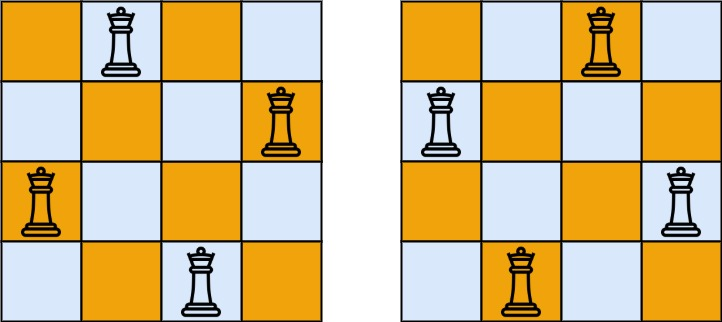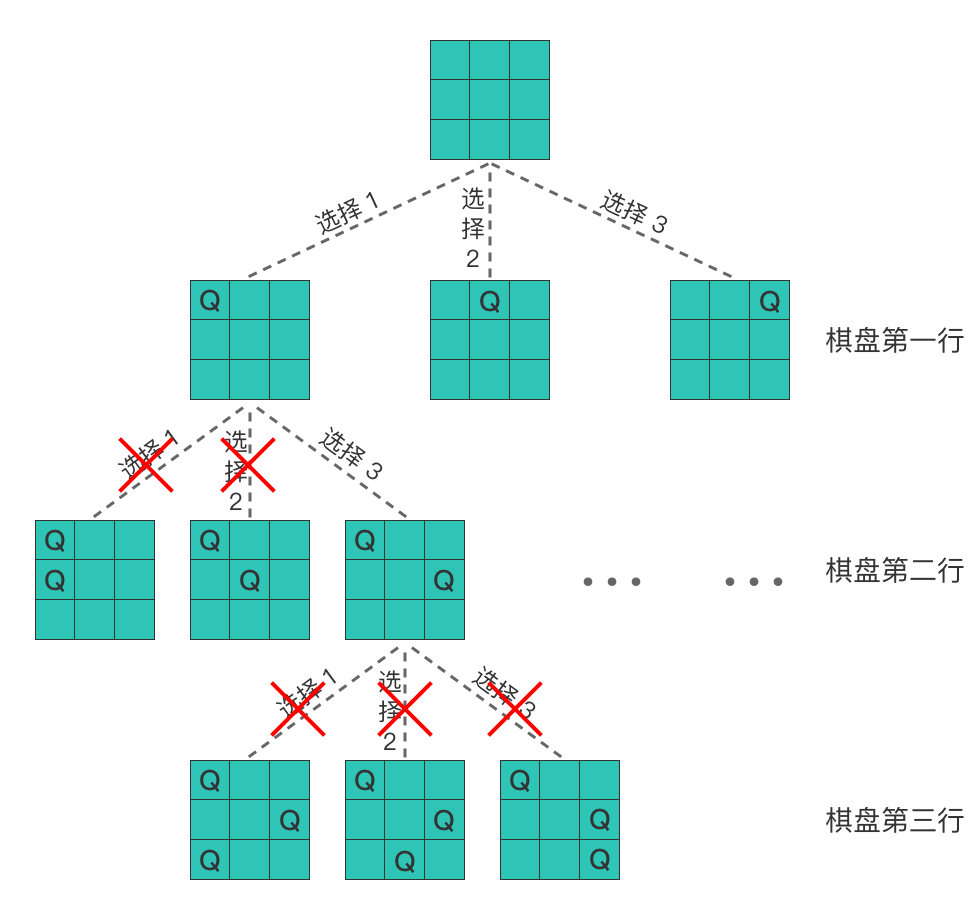0051. N 皇后

# 0051. N 皇后#

• 标签：数组、回溯
• 难度：困难

## 题目大意 #

• n 皇后问题：将 n 个皇后放置在 n * n 的棋盘上，并且使得皇后彼此之间不能攻击。
• 皇后彼此不能相互攻击：指的是任何两个皇后都不能处于同一条横线、纵线或者斜线上。
• $1 \le n \le 9$。

 1 2 3  输入：n = 4 输出：[[".Q..","...Q","Q...","..Q."],["..Q.","Q...","...Q",".Q.."]] 解释：如下图所示，4 皇后问题存在 2 个不同的解法。## 解题思路 #

### 思路 1：回溯算法 #

1. 明确所有选择：根据棋盘中当前行的所有列位置上是否选择放置皇后，画出决策树，如下图所示。

•2. 明确终止条件

• 当遍历到决策树的叶子节点时，就终止了。也就是在最后一行放置完皇后时，递归终止。
3. 将决策树和终止条件翻译成代码：

1. 定义回溯函数：

• 首先我们先使用一个 n * n 大小的二维矩阵 chessboard 来表示当前棋盘，chessboard 中的字符 Q 代表皇后，. 代表空位，初始都为 .
• 然后定义回溯函数 backtrack(chessboard, row):  函数的传入参数是 chessboard（棋盘数组）和 row（代表当前正在考虑放置第 row 行皇后），全局变量是 res（存放所有符合条件结果的集合数组）。
• backtrack(chessboard, row): 函数代表的含义是：在放置好第 row 行皇后的情况下，递归放置剩下行的皇后。
2. 书写回溯函数主体（给出选择元素、递归搜索、撤销选择部分）。

• 枚举出当前行所有的列。对于每一列位置：
• 约束条件：定义一个判断方法，先判断一下当前位置是否与之前棋盘上放置的皇后发生冲突，如果不发生冲突则继续放置，否则则继续向后遍历判断。
• 选择元素：选择 row, col 位置放置皇后，将其棋盘对应位置设置为 Q
• 递归搜索：在该位置放置皇后的情况下，继续递归考虑下一行。
• 撤销选择：将棋盘上 row, col 位置设置为 .
  1 2 3 4 5 6 7 8 9 10 11 12 13 14 15 16 17 18 19 20  # 判断当前位置 row, col 是否与之前放置的皇后发生冲突 def isValid(self, n: int, row: int, col: int, chessboard: List[List[str]]): for i in range(row): if chessboard[i][col] == 'Q': return False i, j = row - 1, col - 1 while i >= 0 and j >= 0: if chessboard[i][j] == 'Q': return False i -= 1 j -= 1 i, j = row - 1, col + 1 while i >= 0 and j < n: if chessboard[i][j] == 'Q': return False i -= 1 j += 1 return True 
 1 2 3 4 5  for col in range(n): # 枚举可放置皇后的列 if self.isValid(n, row, col, chessboard): # 如果该位置与之前放置的皇后不发生冲突 chessboard[row][col] = 'Q' # 选择 row, col 位置放置皇后 backtrack(row + 1, chessboard) # 递归放置 row + 1 行之后的皇后 chessboard[row][col] = '.' # 撤销选择 row, col 位置 
1. 明确递归终止条件（给出递归终止条件，以及递归终止时的处理方法）。
• 当遍历到决策树的叶子节点时，就终止了。也就是在最后一行放置完皇后（即 row == n）时，递归停止。
• 递归停止时，将当前符合条件的棋盘转换为答案需要的形式，然后将其存入答案数组 res 中即可。

### 思路 1：代码 #

  1 2 3 4 5 6 7 8 9 10 11 12 13 14 15 16 17 18 19 20 21 22 23 24 25 26 27 28 29 30 31 32 33 34 35 36 37 38 39 40 41  class Solution: res = [] def backtrack(self, n: int, row: int, chessboard: List[List[str]]): if row == n: temp_res = [] for temp in chessboard: temp_str = ''.join(temp) temp_res.append(temp_str) self.res.append(temp_res) return for col in range(n): if self.isValid(n, row, col, chessboard): chessboard[row][col] = 'Q' self.backtrack(n, row + 1, chessboard) chessboard[row][col] = '.' def isValid(self, n: int, row: int, col: int, chessboard: List[List[str]]): for i in range(row): if chessboard[i][col] == 'Q': return False i, j = row - 1, col - 1 while i >= 0 and j >= 0: if chessboard[i][j] == 'Q': return False i -= 1 j -= 1 i, j = row - 1, col + 1 while i >= 0 and j < n: if chessboard[i][j] == 'Q': return False i -= 1 j += 1 return True def solveNQueens(self, n: int) -> List[List[str]]: self.res.clear() chessboard = [['.' for _ in range(n)] for _ in range(n)] self.backtrack(n, 0, chessboard) return self.res 

### 思路 1：复杂度分析 #

• 时间复杂度：$O(n!)$，其中 $n$ 是皇后数量。
• 空间复杂度：$O(n^2)$，其中 $n$ 是皇后数量。递归调用层数不会超过 $n$，每个棋盘的空间复杂度为 $O(n^2)$，所以空间复杂度为 $O(n^2)$。